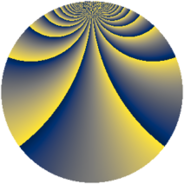# Properties

 Label 690.2.nLevel $690$ Weight $2$ Character orbit 690.n Rep. character $\chi_{690}(89,\cdot)$ Character field $\Q(\zeta_{22})$ Dimension $480$ Newform subspaces $2$ Sturm bound $288$ Trace bound $2$

# Related objects

## Defining parameters

 Level: $$N$$ $$=$$ $$690 = 2 \cdot 3 \cdot 5 \cdot 23$$ Weight: $$k$$ $$=$$ $$2$$ Character orbit: $$[\chi]$$ $$=$$ 690.n (of order $$22$$ and degree $$10$$) Character conductor: $$\operatorname{cond}(\chi)$$ $$=$$ $$345$$ Character field: $$\Q(\zeta_{22})$$ Newform subspaces: $$2$$ Sturm bound: $$288$$ Trace bound: $$2$$ Distinguishing $$T_p$$: $$17$$

## Dimensions

The following table gives the dimensions of various subspaces of $$M_{2}(690, [\chi])$$.

Total New Old
Modular forms 1520 480 1040
Cusp forms 1360 480 880
Eisenstein series 160 0 160

## Trace form

 $$480q - 48q^{4} + 4q^{6} - 12q^{9} + O(q^{10})$$ $$480q - 48q^{4} + 4q^{6} - 12q^{9} + 22q^{15} - 48q^{16} + 4q^{24} - 24q^{25} + 44q^{30} + 56q^{31} - 12q^{36} - 8q^{46} + 140q^{49} - 18q^{54} - 52q^{55} - 22q^{60} + 88q^{61} - 48q^{64} + 88q^{66} - 204q^{69} - 72q^{70} - 184q^{75} - 88q^{79} + 148q^{81} - 22q^{84} + 44q^{85} + 32q^{94} + 4q^{96} + O(q^{100})$$

## Decomposition of $$S_{2}^{\mathrm{new}}(690, [\chi])$$ into newform subspaces

Label Dim. $$A$$ Field CM Traces $q$-expansion
$$a_2$$ $$a_3$$ $$a_5$$ $$a_7$$
690.2.n.a $$240$$ $$5.510$$ None $$-24$$ $$2$$ $$0$$ $$0$$
690.2.n.b $$240$$ $$5.510$$ None $$24$$ $$-2$$ $$0$$ $$0$$

## Decomposition of $$S_{2}^{\mathrm{old}}(690, [\chi])$$ into lower level spaces

$$S_{2}^{\mathrm{old}}(690, [\chi]) \cong$$ $$S_{2}^{\mathrm{new}}(345, [\chi])$$$$^{\oplus 2}$$